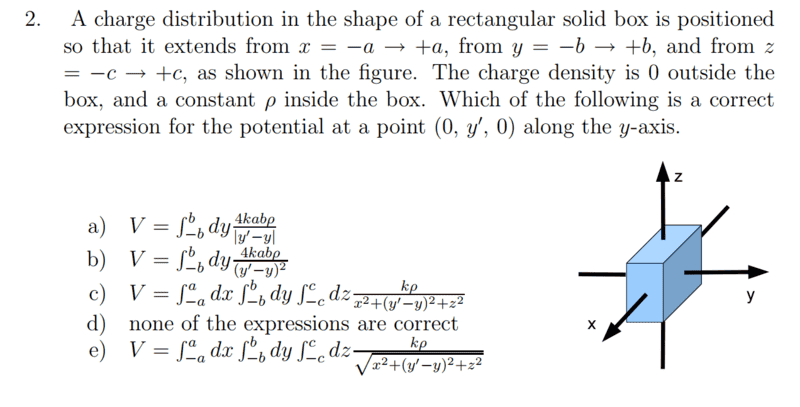# Potential due to Uniform Charge Distribution (3d)

• lorx99
The integral only depends on the limits of the box. In summary, the integral to solve for V(\vec{r}) is given as ## V(\vec{r})= \int \frac{k \rho}{\sqrt{(x-x')^2+(y-y')^2+(z-z')^2}} \, d^3 r' ## and the correct answer is (e). The square root expression is not a constant and can only be integrated over the limits of the box.f

## Homework Statement## Homework Equations

dV= integral(kdQ/dR)

## The Attempt at a Solution

So, I'm familiar with these type of problems but in 2D (like a line of uniform charge).
When the y,z component is added, I'm kinda lost.

i know dQ = p*dV= p*dx*dy*dz. (atleast i think it is).
also the dR = sqrt(x^2 + (y'-y)^2+z^2) since y is the only distance changing.
Now, i get confused as to how to put together the integral. Can someone guide me and help me out?

I am studying for an exam tomorrow :C.

#### Attachments

## V(\vec{r})=\int \frac{k \rho(\vec{r}')}{|\vec{r}-\vec{r}'|}\, d^3 r' ##. Which, if any, of the expressions fit this description? ## \\ ## A hint is they have reversed primes and unprimes, but that is perfectly ok to do. (Take the expression I just gave you and reverse the primes and unprimes). ## \\ ## Also, ## |\vec{r}-\vec{r}'| =\sqrt{(x-x')^2+(y-y')^2+(z-z')^2}##. ## \\ ## Also ## \rho(\vec{r}')=\rho ##. ## \\ ## This one is pretty obvious, but I don't know that I have a simple way to teach you about the limits on triple integrals inside of 10 minutes. Which one do you think might be correct?

Last edited:
## V(\vec{r})=\int \frac{k \rho(\vec{r}')}{|\vec{r}-\vec{r}'|}\, d^3 r' ##. Which, if any, of the expressions fit this description? ## \\ ## A hint is they have reversed primes and unprimes, but that is perfectly ok to do. (Take the expression I just gave you and reverse the primes and unprimes). ## \\ ## Also, ## |\vec{r}-\vec{r}'| =\sqrt{(x-x')^2+(y-y')^2+(z-z')^2}##. ## \\ ## Also ## \rho(\vec{r}')=\rho ##.

How do i deal with the d^3(r)? Also, is the absolute(r-r') just the sqrt(x^2+(y'-y)^2+z^2)?

How do i deal with the d^3(r)? Also, is the absolute(r-r') just the sqrt(x^2+(y'-y)^2+z^2)?
## d^3 r=dx \, dy \, dz ##. It means the same thing. And yes, see my updated post 2. I wrote out ## |r-r' | ## for you. ## \\ ## Let ## \vec{r}=(0,y,0) ##, i.e. ## x=0 ## and ## z=0 ##, and then reverse the primes and unprimes. ## \\ ## I think you might have a pretty good idea which one is the correct answer. I can verify your answer, but as a homework helper, I am not allowed to give you the answer.

Last edited:
## d^3 r=dx \, dy \, dz ##. It means the same thing. And yes, see my updated post 2. I wrote out ## |r-r' | ## for you. ## \\ ## Let ## \vec{r}=(0,y,0) ##, i.e. ## x=0 ## and ## z=0 ##, and then reverse the primes and unprimes. ## \\ ## I think you might have a pretty good idea which one is the correct answer. I can verify your answer, but as a homework helper, I am not allowed to give you the answer.

Ehh. It's not really homework. I'm doing a bunch of practice exams to prepare my exam tomorrow.

So the answer seems to be e) but is the kp/sqrt() just a constant then? and can be taken out of the integrals? The integral only depends on the limits of the box right?

Ehh. It's not really homework. I'm doing a bunch of practice exams to prepare my exam tomorrow.

So the answer seems to be e) but is the kp/sqrt() just a constant then? and can be taken out of the integrals? The integral only depends on the limits of the box right?
The position of the square root on the far right in (e) means that ## x, y, ## and ## z ## get integrated over ## x, y, ## and ## z ##. And no, the square root expression is not a constant. ## \\ ## And the answer is indeed (e). ## \\ ## ## k \rho ## is a constant, so it can go anywhere.

•lorx99